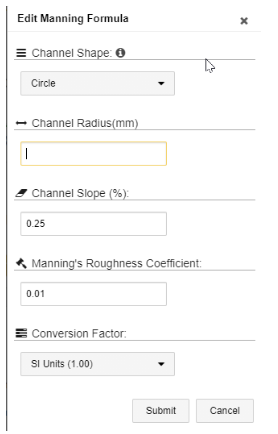# Configuring a Manning Virtual Stream

## The Manning formula is a method of calculating open flow in pipes and channels based on the "Level" measurement.

The Manning formula is a method of calculating open flow in pipes and channels based on the "Level" measurement. A Manning virtual stream is a stream that applies a Manning transformation to its parent stream’s output values (the output values are those appearing in the Final Value column of a stream’s Samples table). The parent stream for the Manning transformation must be a "Level" stream.

One way of calculating open channel flow in pipes and channels without using a flume or weir is the Manning Formula.  Although not as accurate as a hydraulic structure, the formula can provide a sufficient level of accuracy in some applications.

#### Measurement Conditions

For best results when applying the Manning formula, note the following recommendations for the physical characteristics of the pipe:

• The channel should be straight for at least 200 feet (and preferably 1,000 feet).
• The channel should be uniform in cross-section, slope, and roughness.
• There should be no rapids, dips, sudden contractions / expansions, or tributary flows.
• The flow should not be backed up or submerged.

To configure a Manning virtual stream:

1. In the Sites Tree pane, select the device, and then select the LEVEL stream that you want to create a Manning virtual stream for. Note the units of measurement that are used for the stream. In the following example, the metric unit of measurement is used.2. In the right pane, click Configuration & Threshold, and then click Create a Virtual Stream
3. In the Create Transformation window, select Manning as the type, and then click Submit. Refresh the browser page to see the new stream.
4. In the Sites Tree pane, click the new stream, and then click Configuration & Threshold tab. In the Convert to Engineering Units pane, click the pencil icon next to Click to initialize label in order to specify the formula's arguments.The Edit Manning Formula window opens.5.   Set the following parameters:

The unit of measurement for the following parameters depends on the units that were defined in the parent Level stream.

In our example above, the Level stream measures in metrics (mm, mm3, cm, and so forth), so the units here must also be in metrics.

• Channel Shape – Specify either rectangular or circular. Depending on which shape you choose, one of the following parameters is displayed:
• Channel Radius – specify the radius if the channel shape is circular.
• Channel Width – specify the width if the channel shape is rectangular.
• Channel Slope – specify the channel slope at the point of measurement. The slope may be a whole number or a decimal.

Note: Do not convert the slope into a percentage.  For example, if the Slope is 1%, type in 1. Do not type in 0.01 or 1%.

• Manning’s Roughness Coefficient – specify the roughness or friction applied to the flow by the channel. The roughness coefficient can be selected from standard reference roughness based upon the channel / pipe material and its condition.

The following factors might affect the roughness coefficient:

• Surface roughness
• Vegetation
• Silting / scouring
• Obstruction
• Size / shape of channel
• Seasonal change
• Suspended material